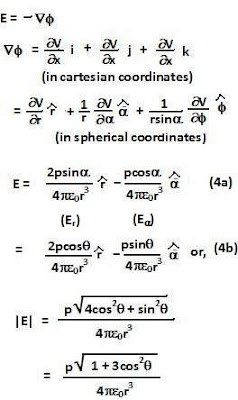## Saturday, June 5, 2010

### Irodov Problem 3.39Let the dipole be represented as a pair of negative and positive charges q and -q located at distance d/2 from the origin as shown in the figure. The electric dipole moment then is given by,I will determine the potential due to the dipole using two different methods. Method 1 is easier.

Method 1: Using basic geometry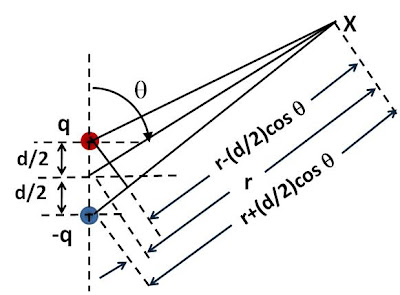When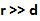, the distance of the point X from the positive and negative charges are respectively, is given byand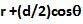as shown in the figure. The potential at point X is given by,Method 2 : Using Taylor's Series
The potential due to these to charges at a point X is then given by,If we assume that d is very very small, then using Taylor's series expansion on the variable d we have,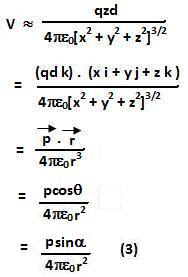The electric field is given by,